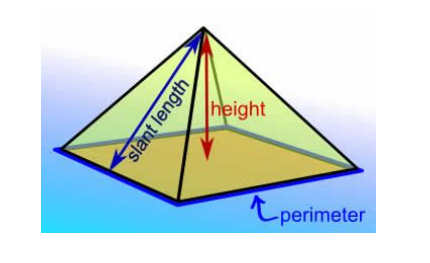# Volume Of A Pyramid

A pyramid is a structure whose outer sides are triangular and converge to a single point on the top. The base of the pyramid can be triangular, quadrilateral or in the shape of any polygon. The most common type of a pyramid is the square pyramid i.e., it has a square base and four triangular faces. Let us discuss the volume of a pyramid in detail.

A typical pyramid looks as follows:## Volume of a Pyramid Formula

Volume is the space enclosed by any close structure.

Calculating the volume of a rectangular pyramid:

Volume of the pyramid can be obtained using the formula given below;

Volume = (B × h)/3 cubic units

Where, B = Area of the base

H = height from base to the tip of the pyramid

### Volume of Pyramid Example

A rectangular pyramid has a height of 12 meters. If the sides of the base measure 4 meters and 6 meters, what is the volume of the pyramid?

Solution:

As, the base is a rectangle, area of the base = 4 × 6 =24 m 2

Volume of the pyramid = (B × h)/3

= (24 × 12)/3 = 288/3

=  96 m 3

Register with BYJU’S – The Learning App and explore more videos to learn with ease.

 Related Links Volume of Cylinder Volume of Cone Volume of Hemisphere Volume of Cube